# PSEB 3rd Class Maths Solutions Chapter 6 Shapes

Punjab State Board PSEB 3rd Class Maths Book Solutions Chapter 6 Shapes Textbook Exercise Questions and Answers.

## PSEB Solutions for Class 3 Maths Chapter 6 Shapes

PAGE 137:

Do you remember?

Question 1.
A joker is given below. Colour it according to given guidelines and count the figures: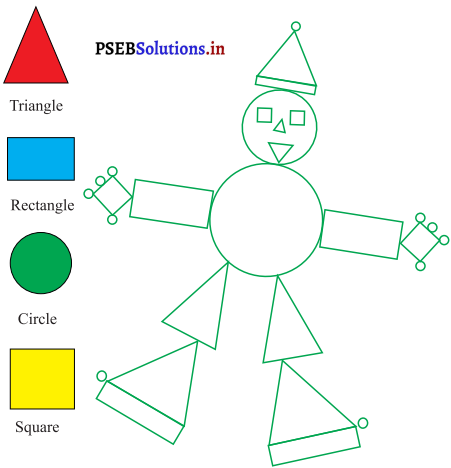Solution.
Do yourself.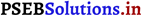See the picture and write:

Question 1.
Number of triangles = ____
Solution.
7

Question 2.
Number of rectangles = ____
Solution.
5

Question 3.
Number of squares = ____
Solution.
4

Question 4.
Number of circles = ____
Solution.
13PAGE 138:

Question 2.
Count and write faces, vertices and edges of the figure gIven below: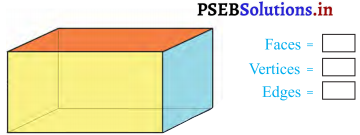Solution.
Faces = 6
Vertices = 8
Edges = 12Question 3.
Name the shapes of the different figures: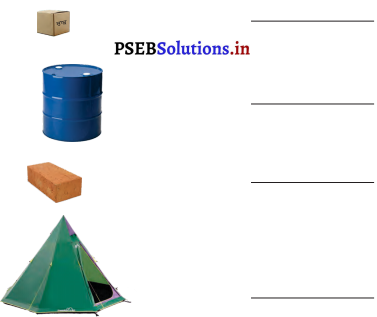Solution.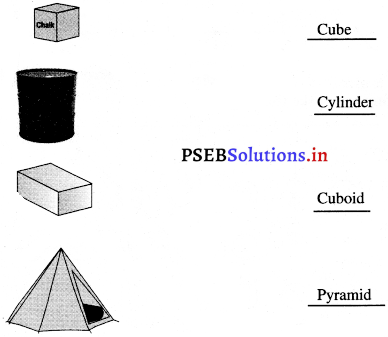Question 4.
Count the triangles: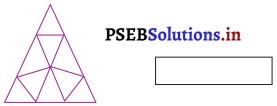Solution.
10

PAGE 141:

Question 1.
Form shapes by joining the dots of the dot grid given below: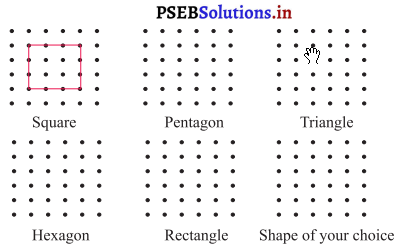Solution.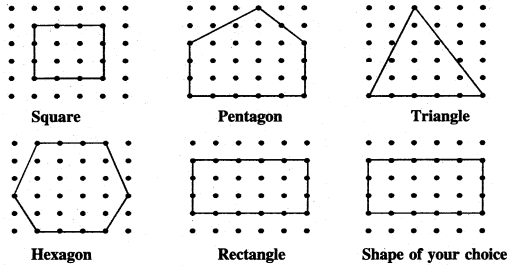Question 2.
Join the counting and make the shape: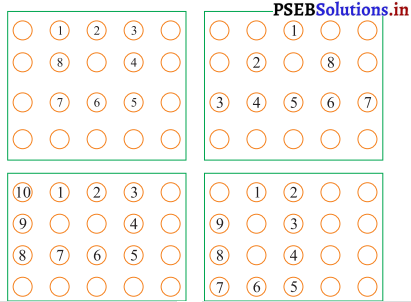Solution.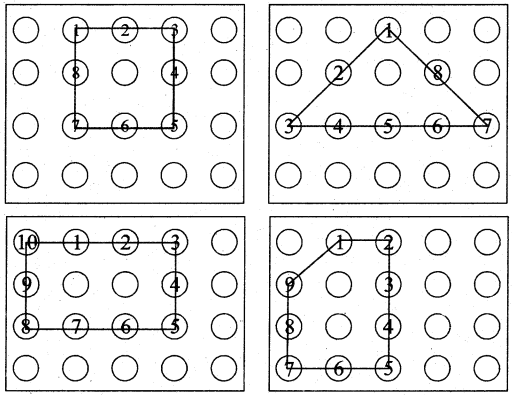PAGE 143:
Let’s do:

Question 1.
Write the sides and vertices of the following 2-D shapes:

(a)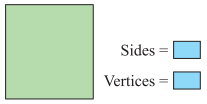Solution.
Sides = 4
Vertices = 4

(b)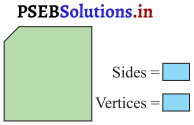Solution.
Sides = 5
Vertices = 5(c)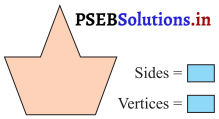Solution.
Sides = 7
Vertices = 7

(d)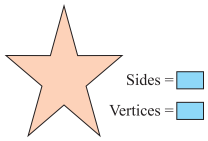Solution.
Sides = 10
Vertices = 10Question 2.
Draw the diagonals in the following figures: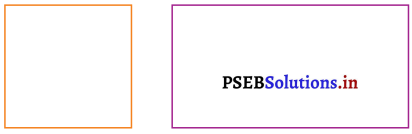Solution.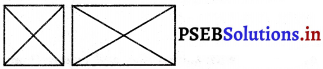PAGE 145:
Let’s do: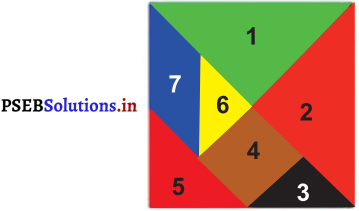Question 1.
How many triangles are there in your tangram?
Solution.
There are five triangles in my tangram.

Question 2.
Make the following shapes by using pieces of tangram.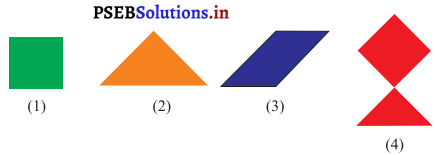Solution.
Draw the following figures by using your tangram.

Question 3.
Try to know that which edge is equal in piece number 2 and 4.
Solution.
Left side of piece number (2) and base of piece number (4) are equal.PAGE 147:

Question 1.
Here, we have two tiles. There are two designs infront of them. Match the design with tile from which it was made.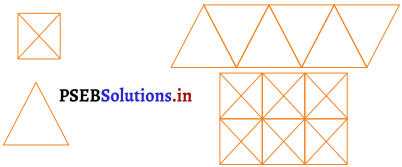Solution.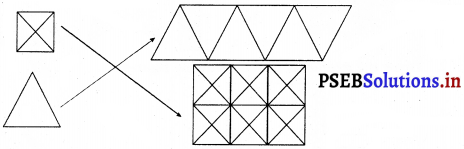PAGE 148:

Question 2.
Cover the following area with shown tiles and colour them.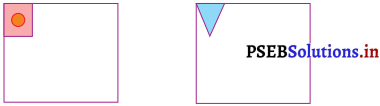Solution.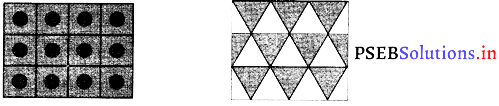Multiple Choice Questions:

Question 1.
Name the figure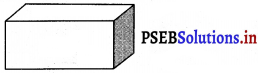(a) Cube
(b) Cuboid
(c) Cone
(d) Cylinder
Solution.
(b) Cuboid.

Question 2.
In the given figure DC is a _______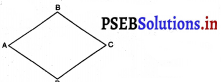(a) Ray
(b) Line
(c) Point
(d) Line Segment.
Solution.
(d) Line Segment.